This site uses cookies. By continuing to browse the ConceptDraw site you are agreeing to our Use of Site Cookies.
ConceptDraw Samples | Computer and Networks — Network Security Diagrams

# Network Security Diagrams

Samples of cybersecurity clipart are created using ConceptDraw DIAGRAM diagramming and vector drawing software enhanced with solution Network Security Diagrams from ConceptDraw Solution Park.

ConceptDraw DIAGRAM provides export of vector graphic multipage documents into multiple file formats: vector graphics (SVG, EMF, EPS), bitmap graphics (PNG, JPEG, GIF, BMP, TIFF), web documents (HTML, PDF), PowerPoint presentations (PPT), Adobe Flash (SWF).

## Tutorials and Solutions:

Video Tutorials: ConceptDraw Solution Park

Solutions: Network Security Diagrams for ConceptDraw DIAGRAM

## Sample 1: 10 Steps to Cyber Security Infographic

Network Security Diagrams sample: Steps to cyber security infographic.

This example is created using ConceptDraw DIAGRAM diagramming and vector drawing software enhanced with Network Security Diagrams solution from ConceptDraw Solution Park.## Sample 2: EFS Operation

Network Security Diagrams sample: EFS operation.

This example is created using ConceptDraw DIAGRAM diagramming and vector drawing software enhanced with Network Security Diagrams solution from ConceptDraw Solution Park.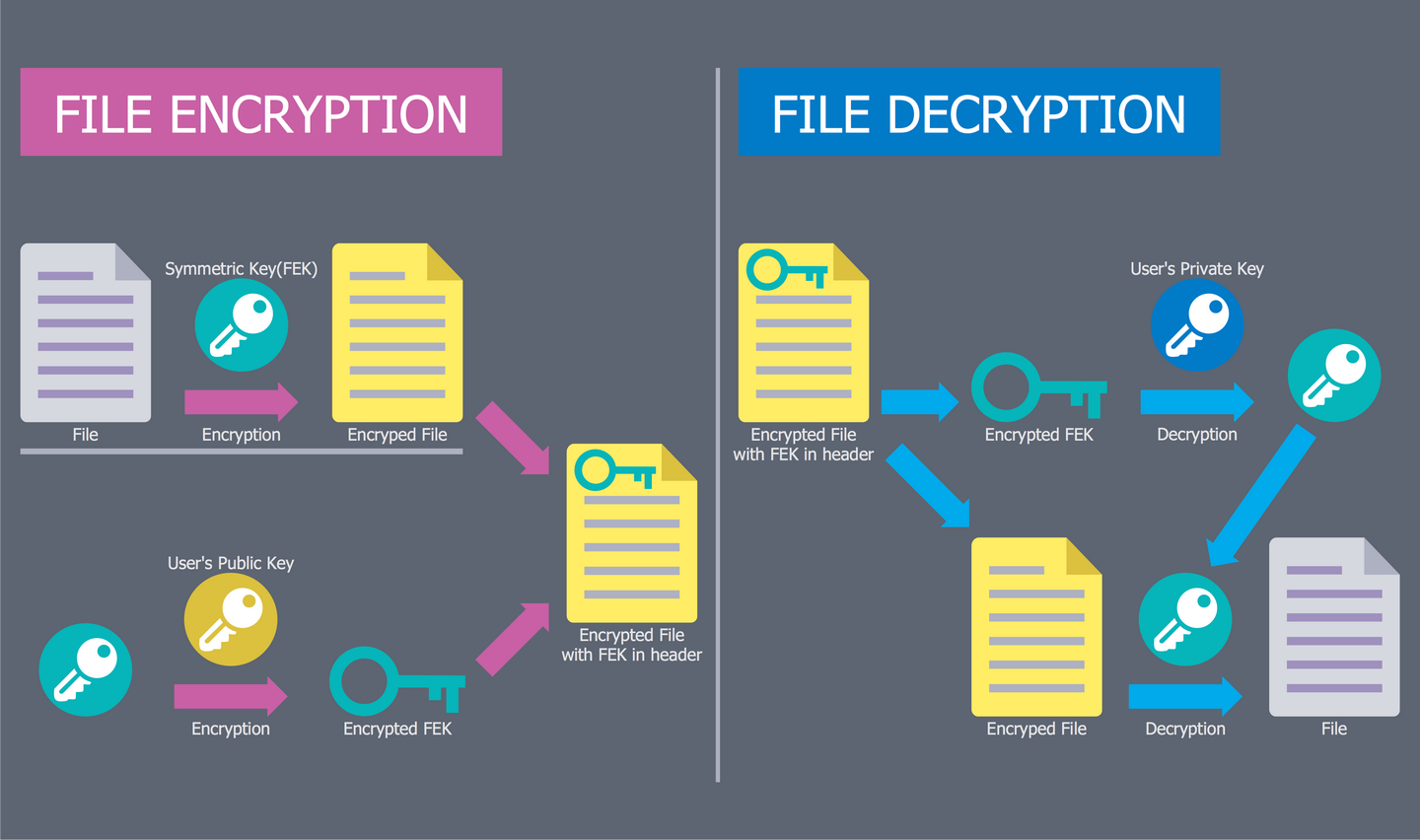## Sample 3: Firewall Between LAN and WAN

Network Security Diagrams sample: Firewall between LAN and WAN.

This example is created using ConceptDraw DIAGRAM diagramming and vector drawing software enhanced with Network Security Diagrams solution from ConceptDraw Solution Park.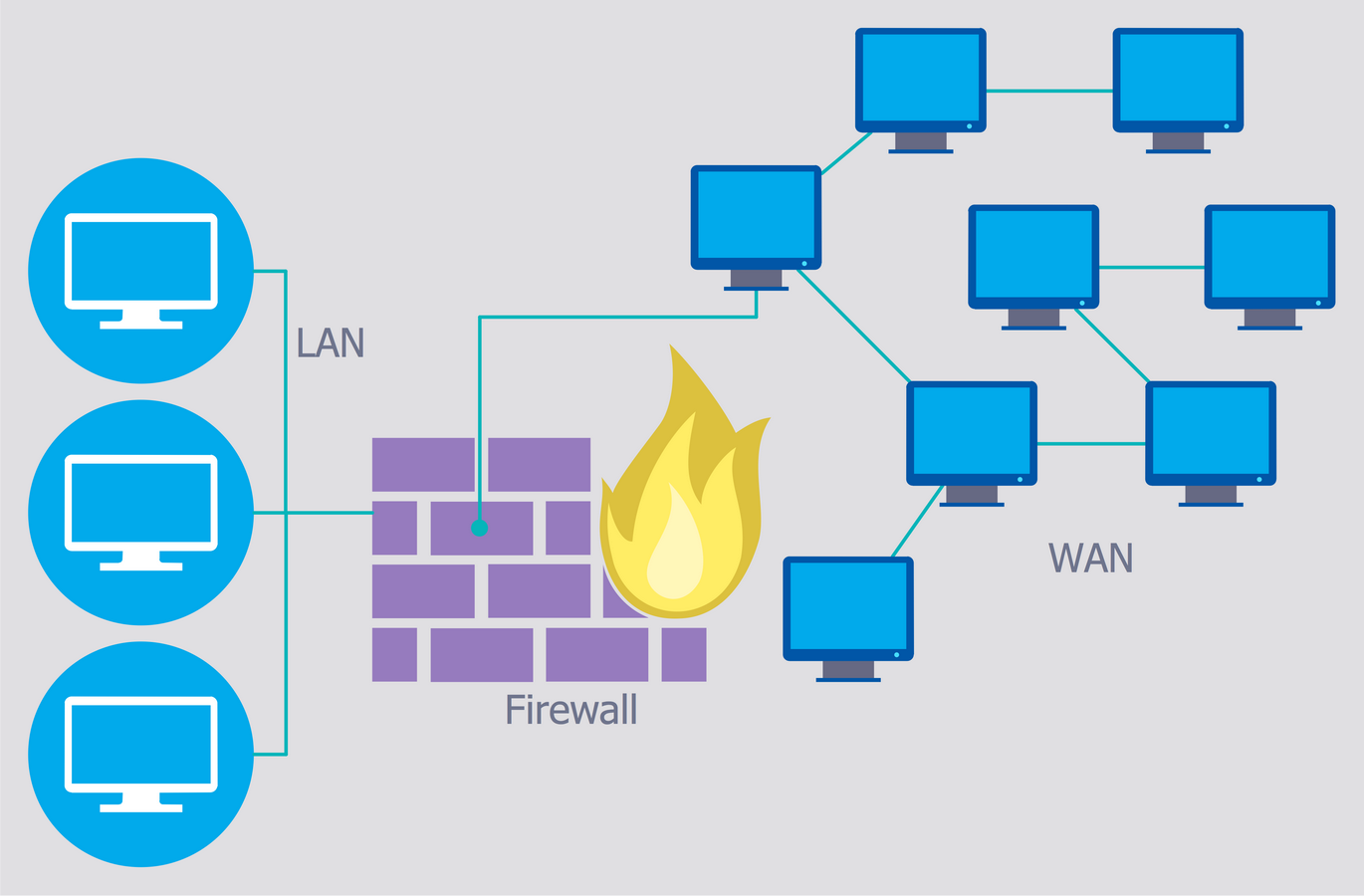## Sample 4: Recommended Network Architecture

Network Security Diagrams sample: Recommended network architecture.

This example is created using ConceptDraw DIAGRAM diagramming and vector drawing software enhanced with Network Security Diagrams solution from ConceptDraw Solution Park.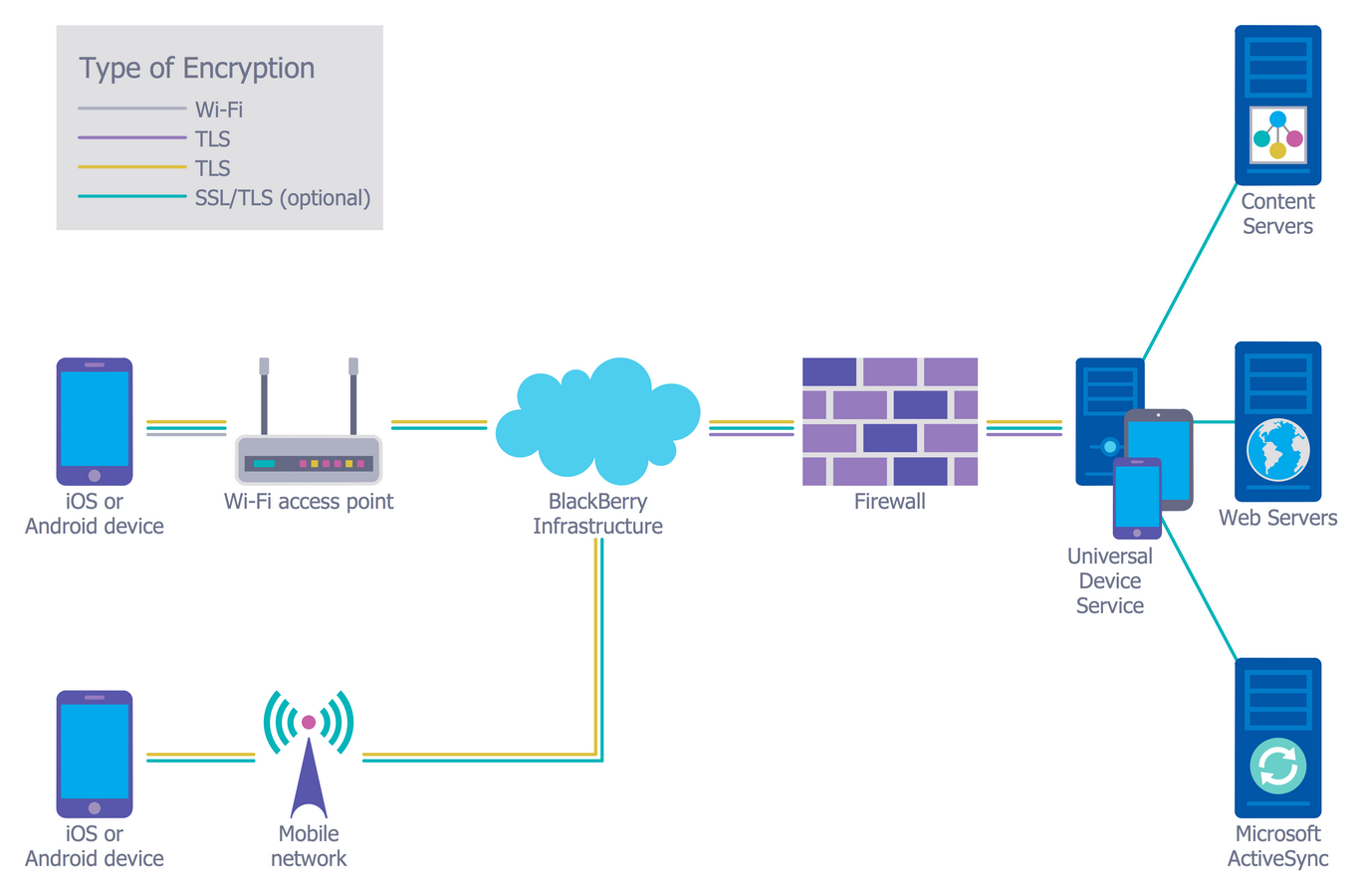## Sample 5: Spread of Conficker Worm

Network Security Diagrams sample: Spread of conficker worm.

This example is created using ConceptDraw DIAGRAM diagramming and vector drawing software enhanced with Network Security Diagrams solution from ConceptDraw Solution Park.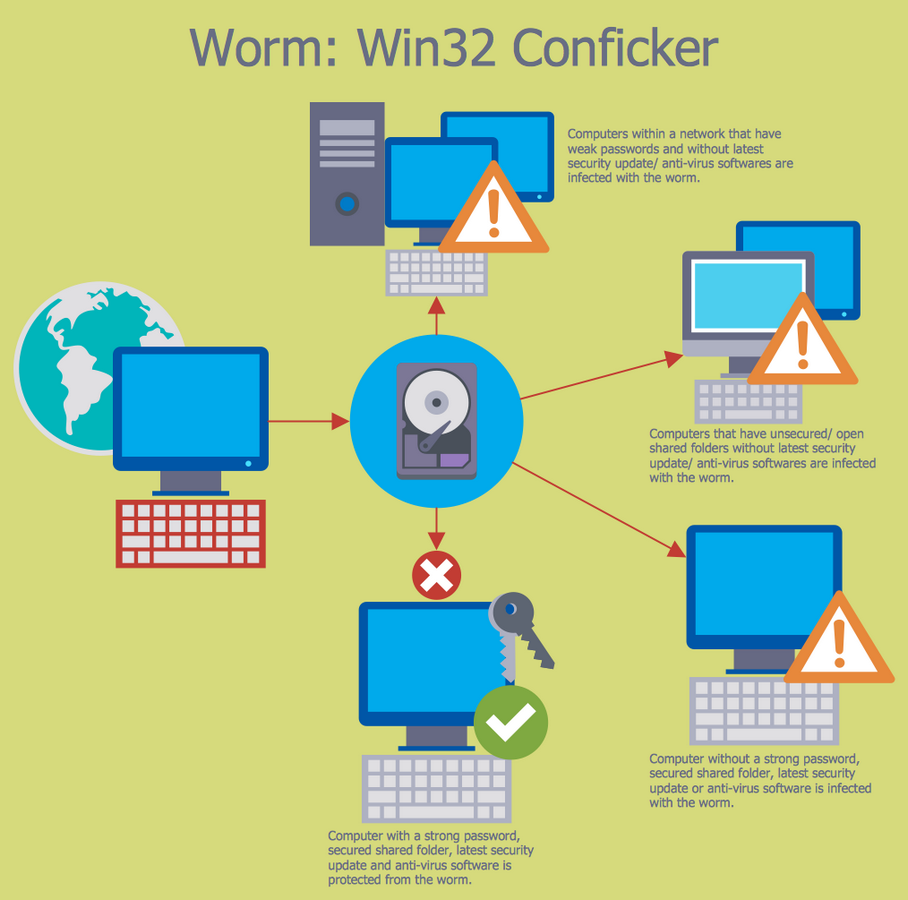## Sample 6: Access Control and Encryption

Network Security Diagrams sample: Access control and encryption.

This example is created using ConceptDraw DIAGRAM diagramming and vector drawing software enhanced with Network Security Diagrams solution from ConceptDraw Solution Park.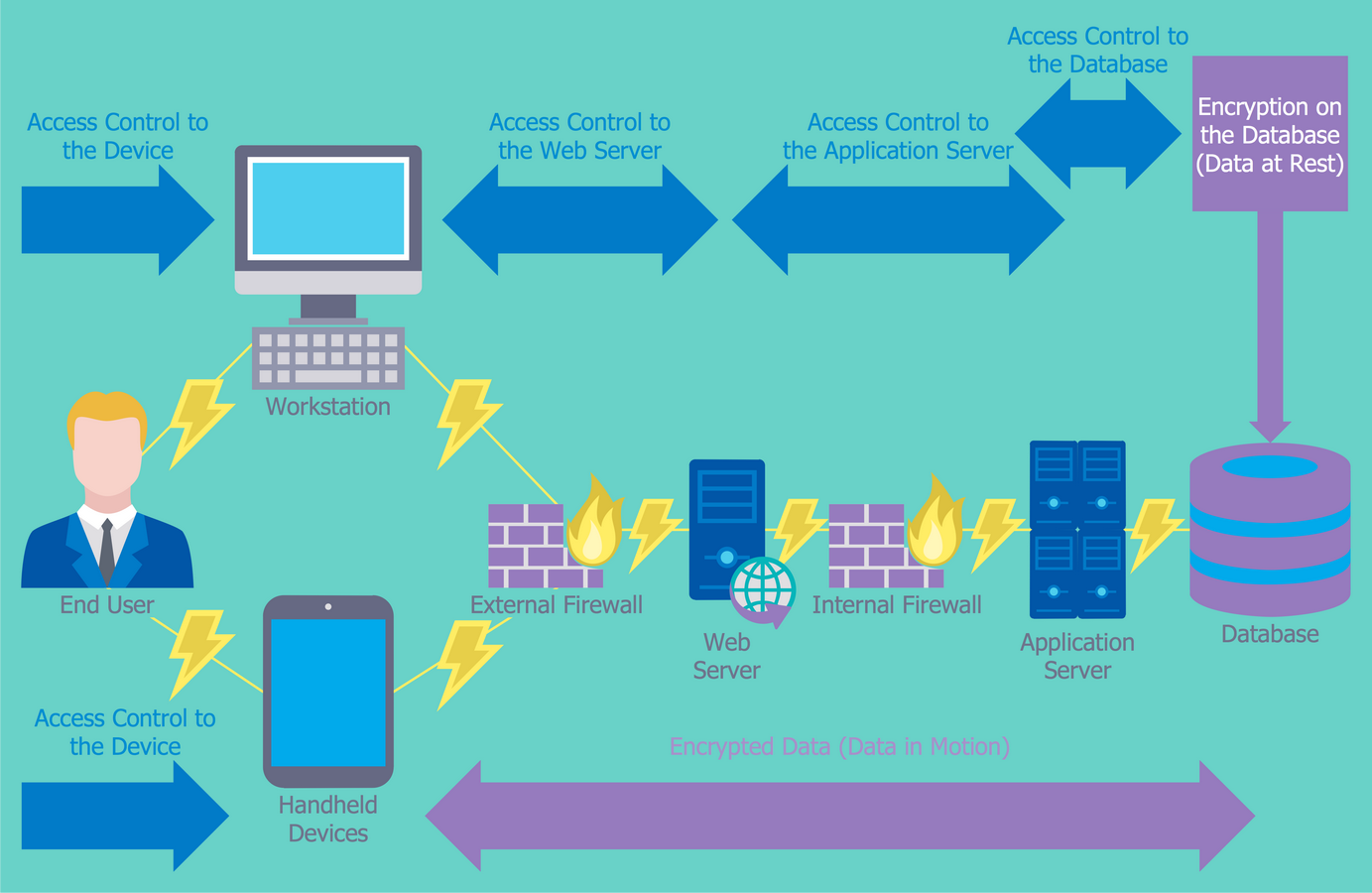## Sample 7: Fork Bomb

Network Security Diagrams sample: Fork bomb.

This example is created using ConceptDraw DIAGRAM diagramming and vector drawing software enhanced with Network Security Diagrams solution from ConceptDraw Solution Park.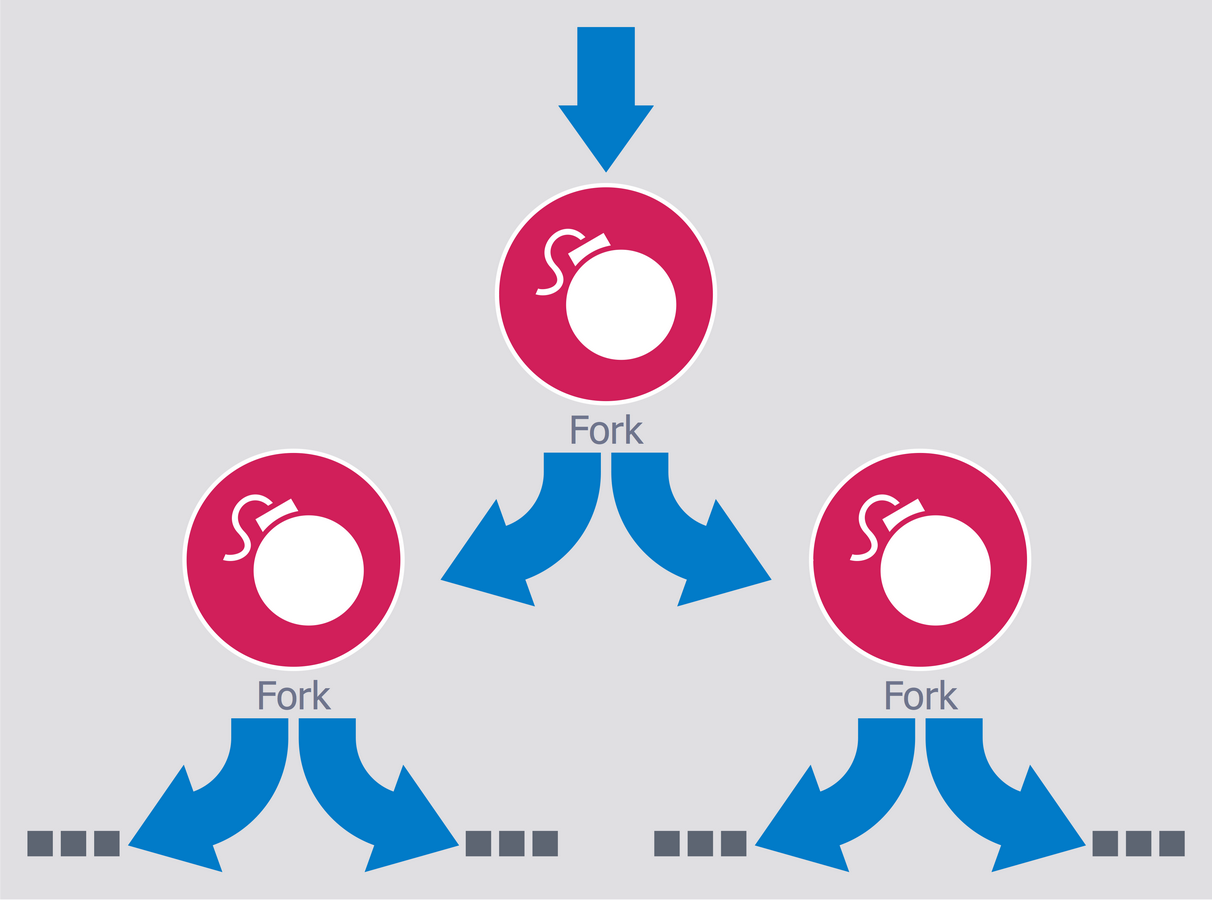## Sample 8: Government Cloud Diagram

Network Security Diagrams sample: Government Cloud diagram.

This example is created using ConceptDraw DIAGRAM diagramming and vector drawing software enhanced with Network Security Diagrams solution from ConceptDraw Solution Park.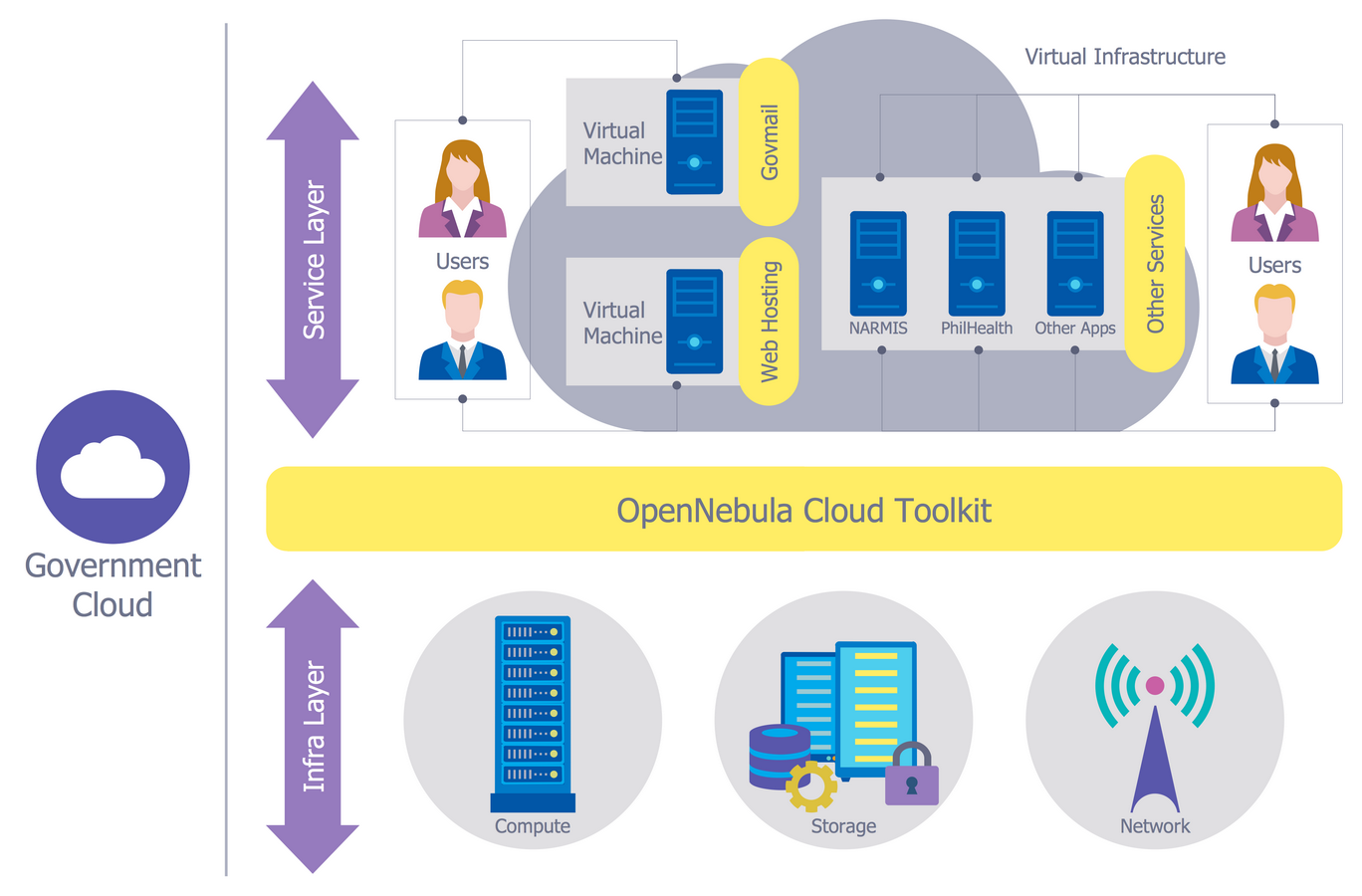## Sample 9: Isolator Architecture Diagram

Network Security Diagrams sample: Isolator architecture diagram.

This example is created using ConceptDraw DIAGRAM diagramming and vector drawing software enhanced with Network Security Diagrams solution from ConceptDraw Solution Park.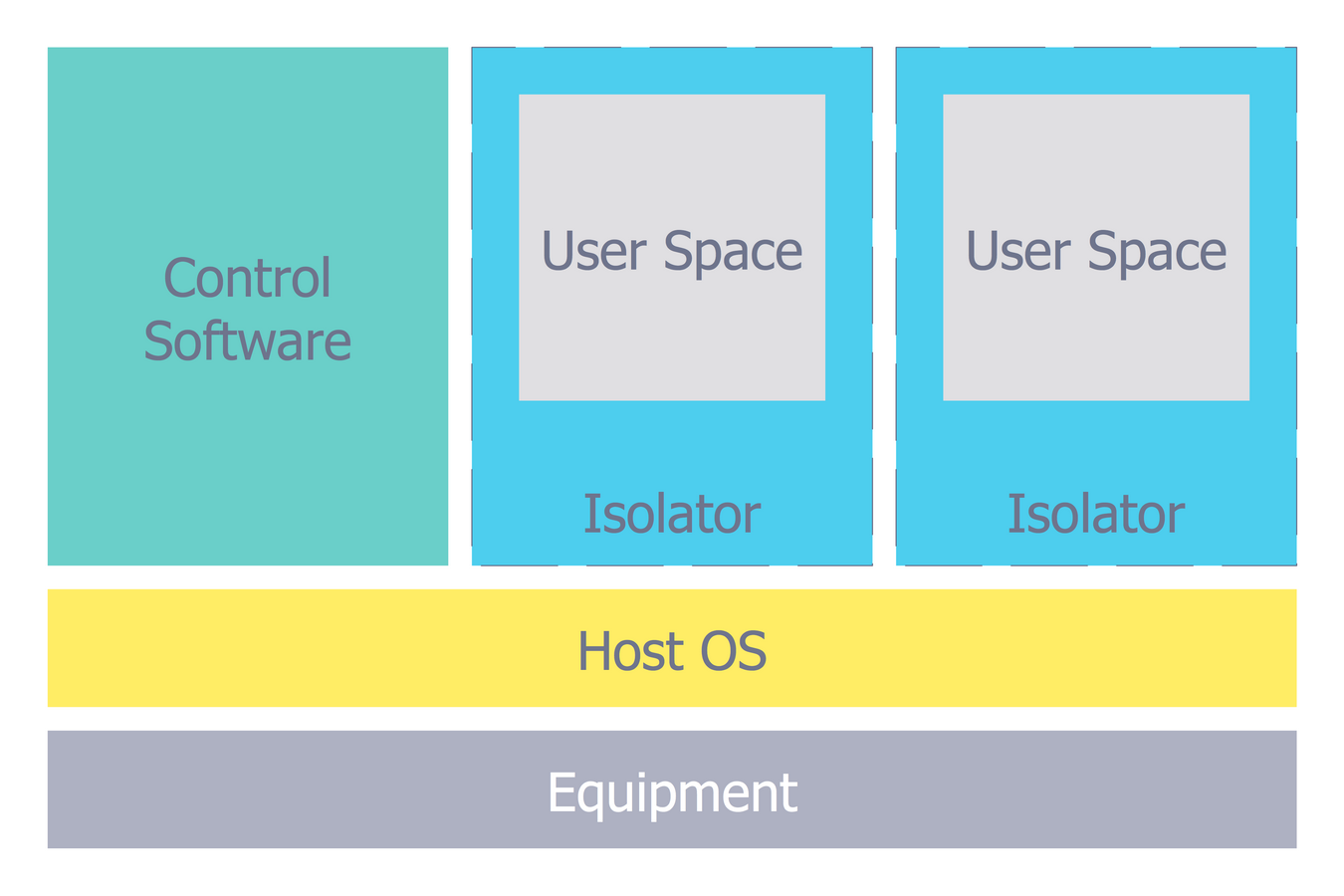## Sample 10: Mobile App Security

Network Security Diagrams sample: Mobile app security.

This example is created using ConceptDraw DIAGRAM diagramming and vector drawing software enhanced with Network Security Diagrams solution from ConceptDraw Solution Park.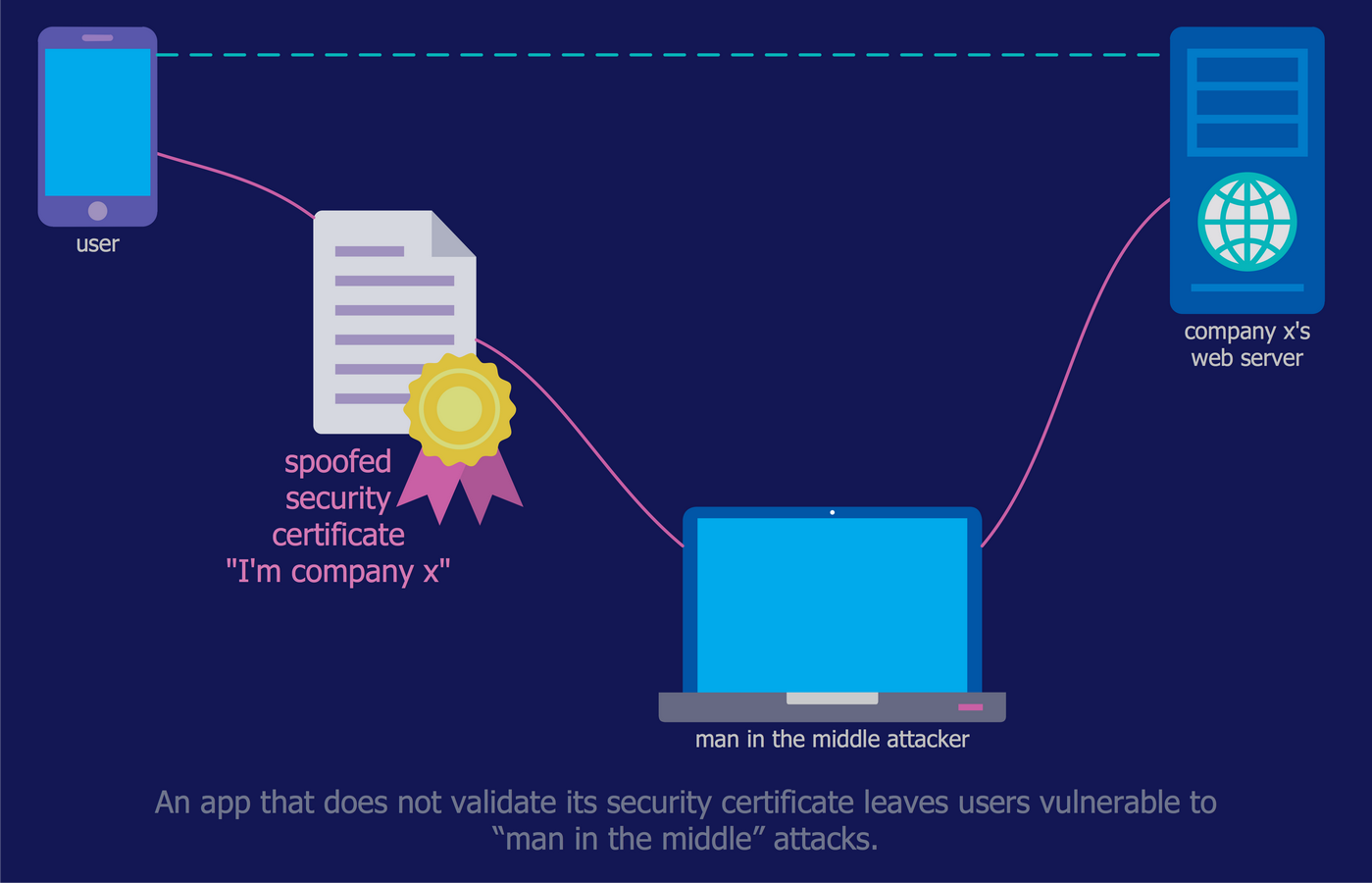All samples are copyrighted CS Odessa's.

Usage of them is covered by Creative Commons “Attribution Non-Commercial No Derivatives” License.

The text you can find at: https://creativecommons.org/licenses/by-nc-nd/3.0